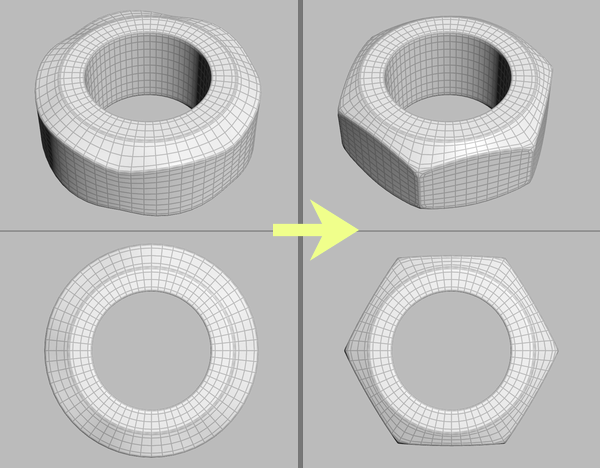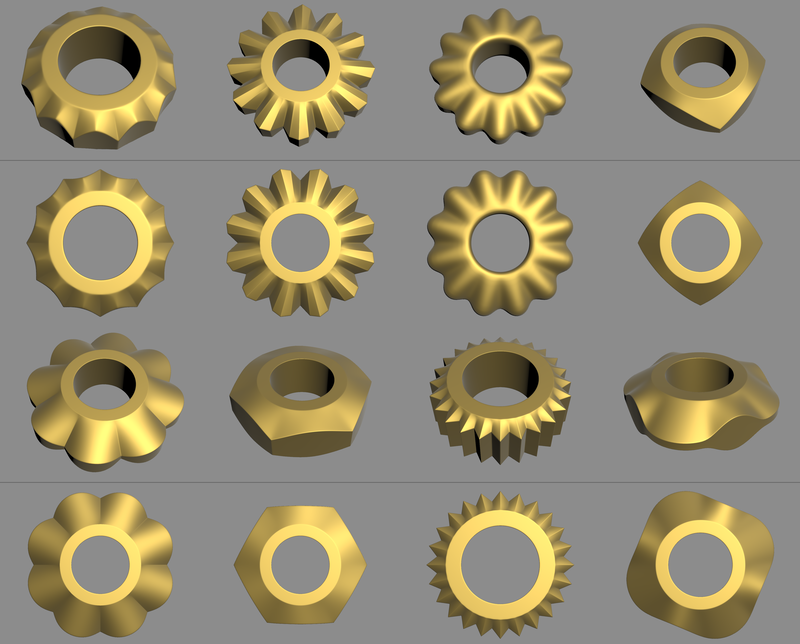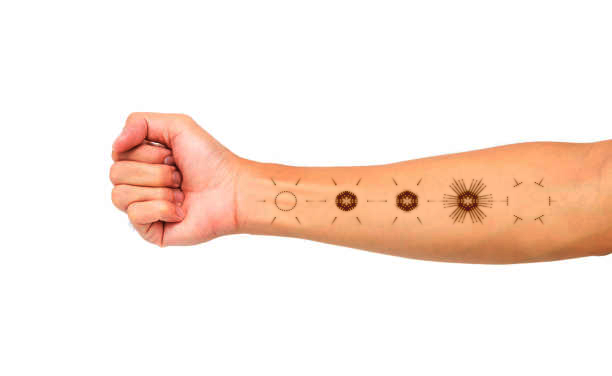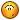## Recommended Posts

Hi, I was shaping a hex nut with VEX when two geometry questions arose:• How can I mathematically define the offset from a circle to an n-gon? Currently I am multiplying by a magic number: sin(u_mod) * 0.12.
• How can I multiply vectors so they end up on an infinite plane defined by a position and a normal? Kind of what planepointdistance() does, but rather intersecting the plane with the points vector than just returning the closest position on the plane. Also I would not want to shoot rays around but rather set this mathematically.

Here is how I deform the tubes outer points at the moment:

```float u = atan(v@P.z, v@P.x);
float u_mod = abs(sin(u * 3));
float u_fit = fit01(u_mod, 0.75, 1.0);

vector nml_flat = normalize(v@N * {1, 0, 1});
v@P -= nml_flat * sin(u_mod) * 0.12;
v@P.y *= u_fit;```

Thank you!

Edited by konstantin magnus

##### Share on other sites

I exposed some parameters, in case someone is looking for a screw nut / cog wheel generator ; )hex_nut.hiplc

•3

##### Share on other sites
4 hours ago, konstantin magnus said:

How can I multiply vectors so they end up on an infinite plane defined by a position and a normal?

By "multiplying" you mean projecting them onto a plane? If so, cross() seems to be enough:

```// P is a vector in world space and POS, NORM are point
// and (unit) normal vector defining infinite plane
vector p0 = P - POS;
vector projected  = cross(p0, NORM);
projected  = cross(NORM, projected) + POS;```

•1

##### Share on other sites
2 hours ago, symek said:

By "multiplying" you mean projecting them onto a plane

Thank you! And yes, by 'multiplying vectors' I was thinking of extending or shortening them, not thinking of using cross products here. So this is a visual summary in 5 steps:

1. When we want to project the grey points from the inner circle on the plane of the outer points and their normals (turqoise),
2. we aim in the opposite direction facing away from the plane points by subtracting circle point positions from the plane positions (yellow),
3. create rectangular vectors to the plane normals by calculating a cross product of the aim direction and the plane points normals (red),
4. and build the cross product of the planes normals and the rectangular directions while adding the planes position (green).
5. When applying this result to the circle points positions, all points are projected on their targeted planes.
```int    pt_plane  = nearpoint(1, v@P);
vector pos_plane = point(1, 'P', pt_plane);
vector nml_plane = point(1, 'N', pt_plane);

v@dir = v@P - pos_plane; // yellow
v@rect = cross(v@dir, nml_plane); // red
v@proj = cross(nml_plane, v@rect) + pos_plane; // green

v@P = v@proj;```

project_on_plane.hiplc

•1

##### Share on other sites

Oh man! You did art with those vectors. I'm jealous!Edited by symek
•1

##### Share on other sites

Well, there wouldnt been a single line without your brain ; )

##### Share on other sites

Hi,

nice tool!

I've modified your example a bit avoiding using sinus but projecting the vectors onto planes instead.

hex_nutX.hipnc

##### Share on other sites

One question remains: How would one scale radial vectors so a circle turns into an n-gon?##### Share on other sites

I'm quite fond of this function:
http://www.sidefx.com/docs/houdini/vex/functions/planepointdistance.html

pretty sure you would be able to use it in your case(not completely sure how it will operate at the corners, but on the straight bits I imagine it should work pretty well)

##### Share on other sites
On 3/10/2019 at 2:55 PM, konstantin magnus said:

One question remains: How would one scale radial vectors so a circle turns into an n-gon?

```int   num_faces = chi("num_faces");

int    nface = floor((float)@ptnum * num_faces / @numpt);
float  alpha = M_PI * (1+2*nface) / num_faces;
int    pin   = nface * @numpt  / num_faces;

vector nml   = set(cos(alpha), 0, -sin(alpha));
vector pos   = point(0, "P", pin);

@P = @P - pos;
@P = cross(@P, nml);
@P = cross(nml, @P) + pos;```

Those divisions by num_faces distract me, but I can't do better## Create an account

Register a new account